# Hedgecraft Part 1: Building a Minimally Correlated Portfolio with Data Science¶

What is the optimal way of constructing a portfolio? A portfolio that (1) consistently generates wealth while minimizing potential lossess and (2) is robust against large market fluctuations and economic downturns? I will explore these questions in some depth in a three-part Hedgecraft series. While the series is aimed at a technical audience, my intentions are to break the technical concepts down into digestible bite-sized pieces suitable for a general audience, institutional investors, and others alike. The approach documented in this notebook (to my knowledge) is novel. To this end, the penultimate goal of the project is an end-to-end FinTech/Portfolio Management product.

## Summary¶

Using insights from Network Science, we build a centrality-based risk model for generating portfolio asset weights. The model is trained with the daily prices of 31 stocks from 2006-2014 and validated in years 2015, 2016, and 2017. As a benchmark, we compare the model with a portolfio constructed with Modern Portfolio Theory (MPT). Our proposed asset allocation algorithm significantly outperformed both the DIJIA and S&P500 indexes in every validation year with an average annual return rate of 38.7%, a 18.85% annual volatility, a 1.95 Sharpe ratio, a -12.22% maximum drawdown, a return over maximum drawdown of 9.75, and a growth-risk-ratio of 4.32. In comparison, the MPT portfolio had a 9.64% average annual return rate, a 16.4% annual standard deviation, a Sharpe ratio of 0.47, a maximum drawdown of -20.32%, a return over maximum drawdown of 1.5, and a growth-risk-ratio of 0.69.

## Background¶

In this series we play the part of an Investment Data Scientist at Bridgewater Associates performing a go/no go analysis on a new idea for risk-weighted asset allocation. Our aim is to develop a network-based model for generating asset weights such that the probability of losing money in any given year is minimized. We've heard down the grapevine that all go-descisions will be presented to Dalio's inner circle at the end of the week and will likely be subject to intense scrutiny. As such, we work with a few highly correlated assets with strict go/no go criteria. We build the model using the daily prices of each stock (with a few replacements*) in the Dow Jones Industrial Average (DJIA). If our recommended portfolio either (1) loses money in any year, (2) does not outperform the market every year, or (3) does not outperform the MPT portfolio---the decision is no go.

• We replaced Visa (V), DowDuPont (DWDP), and Walgreens (WBA) with three alpha generators: Google (GOOGL), Amazon (AMZN), and Altaba (AABA) and, for the sake of model building, one poor performing stock: General Electric (GE). The dataset is found on Kaggle.

### Asset Diversification and Allocation¶

The building blocks of a portfolio are assets (resources with economic value expected to increase over time). Each asset belongs to one of seven primary asset classes: cash, equitiy, fixed income, commodities, real-estate, alternative assets, and more recently, digital (such as cryptocurrency and blockchain). Within each class are different asset types. For example: stocks, index funds, and equity mutual funds all belong to the equity class while gold, oil, and corn belong to the commodities class. An emerging consensus in the financial sector is this: a portfolio containing assets of many classes and types hedges against potential losses by increasing the number of revenue streams. In general the more diverse the portfolio the less likely it is to lose money. Take stocks for example. A diversified stock portfolio contains positions in multiple sectors. We call this asset diversification, or more simply diversification. Below is a table summarizing the asset classes and some of their respective types.

Cash Equity Fixed Income Commodities Real-Estate Alternative Assets Digital
US Dollar US Stocks US Bonds Gold REIT's Structured Credit Cryptocurrencies
Japenese Yen Foreign Stocks Foreign Bonds Oil Commerical Properties Liquidations Security Tokens
Chinese Yaun Index Funds Deposits Wheat Land Aviation Assets Online Stores
UK Pound Mutual Funds Debentures Corn Industrial Properties Collectables Online Media

An investor solves the following (asset allocation) problem: given X dollars and N assets find the best possible way of breaking X into N pieces. By "best possible" we mean maximizing our returns subject to minimizing the risk of our initial investment. In other words, we aim to consistently grow X irrespective of the overall state of the market. In what follows, we explore provocative insights by Ray Dalio and others on portfolio construction.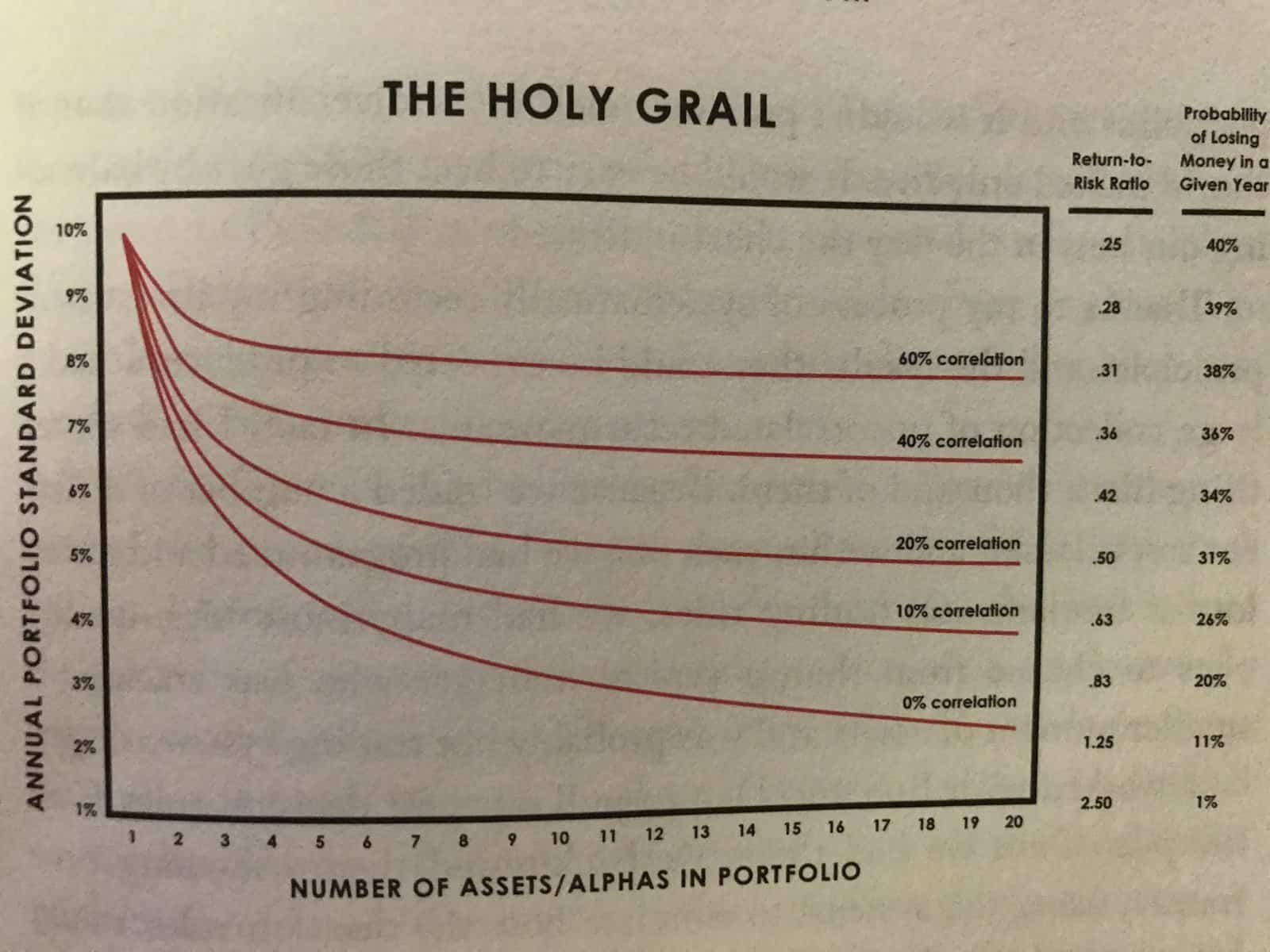Source: Principles by Ray Dalio (Summary)

The above chart depicts the behaviour of a portfolio with increasing diversification. Along the x-axis is the number of asset types. Along the y-axis is how "spread out" the annual returns are. A lower annual standard deviation indicates smaller fluctuations in each revenue stream, and in turn a diminished risk exposure. The "Holy Grail" so to speak, is to (1) find the largest number of assets that are the least correlated and (2) allocate X dollars to those assets such that the probability of losing money any given year is minimized. The underlying principle is this: the portfolio most robust against large market fluctuations and economic downturns is a portfolio with assets that are the most independent of eachother.

# Exploratory Data Analysis and Cleaning¶

Before we dive into the meat of our asset allocation model, we first explore, clean, and preprocess our historical price data for time-series analyses. In this section we complete the following.

• Observe how many rows and columns are in our dataset and what they mean.
• Observe the datatypes of the columns and update them if needed.
• Take note of how the data is structured and what preprocessing will be necessary for time-series analyses.
• Deal with any missing data accordingly.
• Rename the stock tickers to the company names for readability.
In :
#import data manipulation (pandas) and numerical manipulation (numpy) modules
import pandas as pd
import numpy as np

#silence warnings
import warnings
warnings.filterwarnings("ignore")

#reads the csv file into pandas DataFrame

#prints first 5 rows of the DataFrame

Out:
Date Open High Low Close Volume Name
0 2006-01-03 77.76 79.35 77.24 79.11 3117200 MMM
1 2006-01-04 79.49 79.49 78.25 78.71 2558000 MMM
2 2006-01-05 78.41 78.65 77.56 77.99 2529500 MMM
3 2006-01-06 78.64 78.90 77.64 78.63 2479500 MMM
4 2006-01-09 78.50 79.83 78.46 79.02 1845600 MMM
• Date: date (yyyy-mm-dd)
• Open: daily opening prices (USD)
• High: daily high prices (USD)
• Low: daily low prices (USD)
• Close: daily closing prices (USD)
• Volume daily volume (number of shares traded)
• Name: ticker (abbreviates company name)
In :
#prints last 5 rows
df.tail()

Out:
Date Open High Low Close Volume Name
93607 2017-12-22 71.42 71.87 71.22 71.58 10979165 AABA
93608 2017-12-26 70.94 71.39 69.63 69.86 8542802 AABA
93609 2017-12-27 69.77 70.49 69.69 70.06 6345124 AABA
93610 2017-12-28 70.12 70.32 69.51 69.82 7556877 AABA
93611 2017-12-29 69.79 70.13 69.43 69.85 6613070 AABA
In :
#prints information about the DataFrame
df.info()

<class 'pandas.core.frame.DataFrame'>
RangeIndex: 93612 entries, 0 to 93611
Data columns (total 7 columns):
Date      93612 non-null object
Open      93587 non-null float64
High      93602 non-null float64
Low       93592 non-null float64
Close     93612 non-null float64
Volume    93612 non-null int64
Name      93612 non-null object
dtypes: float64(4), int64(1), object(2)
memory usage: 5.0+ MB


Some observations:

• The dataset has 93,612 rows and 7 columns.
• The Date column is not a DateTime object (we need to change this).
• For time-series analyses we need to preprocess the data (we address this in the proceeding section).
• There are missing values in the Open, High, and Low columns (we adress this after preprocessing the data).
• We also want to map the tickers (e.g., MMM) to the company names (e.g., 3M).
• Finally, we need to set the index as the date.
In :
#changes Date column to a DateTime object
df['Date'] = pd.to_datetime(df['Date'])

In :
#prints unique tickers in the Name column
print(df['Name'].unique())

['MMM' 'AXP' 'AAPL' 'BA' 'CAT' 'CVX' 'CSCO' 'KO' 'DIS' 'XOM' 'GE' 'GS'
'HD' 'IBM' 'INTC' 'JNJ' 'JPM' 'MCD' 'MRK' 'MSFT' 'NKE' 'PFE' 'PG' 'TRV'
'UTX' 'UNH' 'VZ' 'WMT' 'GOOGL' 'AMZN' 'AABA']

In :
#dictionary of tickers and their respective company names
ticker_mapping = {'AABA':'Altaba',
'AAPL':'Apple',
'AMZN': 'Amazon',
'AXP':'American Express',
'BA':'Boeing',
'CAT':'Caterpillar',
'MMM':'3M',
'CVX':'Chevron',
'CSCO':'Cisco Systems',
'KO':'Coca-Cola',
'DIS':'Walt Disney',
'XOM':'Exxon Mobil',
'GE': 'General Electric',
'GS':'Goldman Sachs',
'HD': 'Home Depot',
'IBM': 'IBM',
'INTC': 'Intel',
'JNJ':'Johnson & Johnson',
'JPM':'JPMorgan Chase',
'MCD':'Mcdonald\'s',
'MRK':'Merk',
'MSFT':'Microsoft',
'NKE':'Nike',
'PFE':'Pfizer',
'PG':'Procter & Gamble',
'TRV':'Travelers',
'UTX':'United Technologies',
'UNH':'UnitedHealth',
'VZ':'Verizon',
'WMT':'Walmart',

#changes the tickers in df to the company names
df['Name'] = df['Name'].map(ticker_mapping)

In :
#sets the Date column as the index
df.set_index('Date', inplace=True)


# Preprocessing for Time-Series Analysis¶

In this section we do the following.

1. Break the data in two pieces: historical prices from 2006-2014 (df_train) and from 2015-2017 (df_validate). We build our model portfolio using the former and test it with the latter.

2. We add a column to df_train recording the difference between the daily closing and opening prices Close_diff.

3. We create a seperate DataFrame for the Open, High, Low, Close, and Close_diff time-series.
• Pivot the tickers in the Name column of df_train to the column names of the above DataFrames and set the values as the daily prices

4. Transform each time-series so that it's stationary.
• We do this by detrending with the pd.diff() method

5. Finally, remove the missing data.
In :
#traning dataset
df_train = df.loc['2006-01-03':'2015-01-01']
df_train.tail()

Out:
Open High Low Close Volume Name
Date
2014-12-24 50.19 50.92 50.19 50.65 5962870 Altaba
2014-12-26 50.65 51.06 50.61 50.86 5170048 Altaba
2014-12-29 50.67 51.01 50.51 50.53 6624489 Altaba
2014-12-30 50.35 51.27 50.35 51.22 10703455 Altaba
2014-12-31 51.54 51.68 50.46 50.51 9305013 Altaba
In :
#testing dataset
df_validate = df.loc['2015-01-01':'2017-12-31']
df_validate.tail()

Out:
Open High Low Close Volume Name
Date
2017-12-22 71.42 71.87 71.22 71.58 10979165 Altaba
2017-12-26 70.94 71.39 69.63 69.86 8542802 Altaba
2017-12-27 69.77 70.49 69.69 70.06 6345124 Altaba
2017-12-28 70.12 70.32 69.51 69.82 7556877 Altaba
2017-12-29 69.79 70.13 69.43 69.85 6613070 Altaba

It's always a good idea to check we didn't lose any data after the split.

In :
#returns True if no data was lost after the split and False otherwise.
df_train.shape + df_validate.shape == df.shape

Out:
True
In :
# sets each column as a stock and every row as a daily closing price
df_validate = df_validate.pivot(columns='Name', values='Close')

In :
df_validate.head()

Out:
Name 3M Altaba Amazon American Express Apple Boeing Caterpillar Chevron Cisco Systems Coca-Cola ... Microsoft Nike Pfizer Procter & Gamble Travelers United Technologies UnitedHealth Verizon Walmart Walt Disney
Date
2015-01-02 164.06 50.17 308.52 93.02 109.33 129.95 91.88 112.58 27.61 42.14 ... 46.76 47.52 31.33 90.44 105.44 115.04 100.78 46.96 85.90 93.75
2015-01-05 160.36 49.13 302.19 90.56 106.25 129.05 87.03 108.08 27.06 42.14 ... 46.32 46.75 31.16 90.01 104.17 113.12 99.12 46.57 85.65 92.38
2015-01-06 158.65 49.21 295.29 88.63 106.26 127.53 86.47 108.03 27.05 42.46 ... 45.65 46.48 31.42 89.60 103.24 111.52 98.92 47.04 86.31 91.89
2015-01-07 159.80 48.59 298.42 90.30 107.75 129.51 87.81 107.94 27.30 42.99 ... 46.23 47.44 31.85 90.07 105.00 112.73 99.93 46.19 88.60 92.83
2015-01-08 163.63 50.23 300.46 91.58 111.89 131.80 88.71 110.41 27.51 43.51 ... 47.59 48.53 32.50 91.10 107.18 114.65 104.70 47.18 90.47 93.79

5 rows × 31 columns

In :
#creates a new column with the difference beteween the closing and opening prices
df_train['Close_Diff'] = df_train.loc[:,'Close'] - df_train.loc[:,'Open']

In :
df_train.head()

Out:
Open High Low Close Volume Name Close_Diff
Date
2006-01-03 77.76 79.35 77.24 79.11 3117200 3M 1.35
2006-01-04 79.49 79.49 78.25 78.71 2558000 3M -0.78
2006-01-05 78.41 78.65 77.56 77.99 2529500 3M -0.42
2006-01-06 78.64 78.90 77.64 78.63 2479500 3M -0.01
2006-01-09 78.50 79.83 78.46 79.02 1845600 3M 0.52
In :
#creates a DataFrame for each time-series (see In )
df_train_close = df_train.pivot(columns='Name', values='Close')
df_train_open = df_train.pivot(columns='Name', values='Open')
df_train_close_diff = df_train.pivot(columns='Name', values='Close_Diff')
df_train_high = df_train.pivot(columns='Name', values='High')
df_train_low = df_train.pivot(columns='Name', values='Low')

#makes a copy of the traning dataset
df_train_close_copy = df_train_close.copy()


Out:
Name 3M Altaba Amazon American Express Apple Boeing Caterpillar Chevron Cisco Systems Coca-Cola ... Microsoft Nike Pfizer Procter & Gamble Travelers United Technologies UnitedHealth Verizon Walmart Walt Disney
Date
2006-01-03 79.11 40.91 47.58 52.58 10.68 70.44 57.80 59.08 17.45 20.45 ... 26.84 10.74 23.78 58.78 45.99 56.53 61.73 30.38 46.23 24.40
2006-01-04 78.71 40.97 47.25 51.95 10.71 71.17 59.27 58.91 17.85 20.41 ... 26.97 10.69 24.55 58.89 46.50 56.19 61.88 31.27 46.32 23.99
2006-01-05 77.99 41.53 47.65 52.50 10.63 70.33 59.27 58.19 18.35 20.51 ... 26.99 10.76 24.58 58.70 46.95 55.98 61.69 31.63 45.69 24.41
2006-01-06 78.63 43.21 47.87 52.68 10.90 69.35 60.45 59.25 18.77 20.70 ... 26.91 10.72 24.85 58.64 47.21 56.16 62.90 31.35 45.88 24.74
2006-01-09 79.02 43.42 47.08 53.99 10.86 68.77 61.55 58.95 19.06 20.80 ... 26.86 10.88 24.85 59.08 47.23 56.80 61.40 31.48 45.71 25.00

5 rows × 31 columns

## Detrending and Additional Data Cleaning¶

In :
#creates a list of stocks
stocks = df_train_close.columns.tolist()

#list of training DataFrames containing each time-series
df_train_list = [df_train_close, df_train_open, df_train_close_diff, df_train_high, df_train_low]

#detrends each time-series for each DataFrame
for df in df_train_list:
for s in stocks:
df[s] = df[s].diff()

In :
df_train_close.head()

Out:
Name 3M Altaba Amazon American Express Apple Boeing Caterpillar Chevron Cisco Systems Coca-Cola ... Microsoft Nike Pfizer Procter & Gamble Travelers United Technologies UnitedHealth Verizon Walmart Walt Disney
Date
2006-01-03 NaN NaN NaN NaN NaN NaN NaN NaN NaN NaN ... NaN NaN NaN NaN NaN NaN NaN NaN NaN NaN
2006-01-04 -0.40 0.06 -0.33 -0.63 0.03 0.73 1.47 -0.17 0.40 -0.04 ... 0.13 -0.05 0.77 0.11 0.51 -0.34 0.15 0.89 0.09 -0.41
2006-01-05 -0.72 0.56 0.40 0.55 -0.08 -0.84 0.00 -0.72 0.50 0.10 ... 0.02 0.07 0.03 -0.19 0.45 -0.21 -0.19 0.36 -0.63 0.42
2006-01-06 0.64 1.68 0.22 0.18 0.27 -0.98 1.18 1.06 0.42 0.19 ... -0.08 -0.04 0.27 -0.06 0.26 0.18 1.21 -0.28 0.19 0.33
2006-01-09 0.39 0.21 -0.79 1.31 -0.04 -0.58 1.10 -0.30 0.29 0.10 ... -0.05 0.16 0.00 0.44 0.02 0.64 -1.50 0.13 -0.17 0.26

5 rows × 31 columns

In :
#counts the missing values in each column
df_train_close.isnull().sum()

Out:
Name
3M                     1
Altaba                 3
Amazon                 3
American Express       1
Apple                  3
Boeing                 1
Caterpillar            1
Chevron                1
Cisco Systems          3
Coca-Cola              1
Exxon Mobil            1
General Electric       1
Goldman Sachs          1
Home Depot             1
IBM                    1
Intel                  3
JPMorgan Chase         1
Johnson & Johnson      1
Mcdonald's             1
Merk                   3
Microsoft              3
Nike                   1
Pfizer                 1
Procter & Gamble       1
Travelers              1
United Technologies    1
UnitedHealth           1
Verizon                1
Walmart                1
Walt Disney            1
dtype: int64

Since the number of missing values in every column is considerably less than 1% of the total number of rows (93,612) we can safely drop them.

In :
#drops all missing values in each DataFrame
for df in df_train_list:
df.dropna(inplace=True)


# Building an Asset Correlation Network¶

Now that the data is preprocessed we can start thinking our way through the problem creatively. To refresh our memory, let's restate the problem.

Given the $N$ assets in our portfolio, find a way of computing the allocation weights $w_{i}$, $\Big( \sum_{i=1}^{N}w_{i}=1\Big)$ such that assets more correlated with each other obtain lower weights while those less correlated with each other obtain higher weights.

One way of tackling the above is to think of our portfolio as a weighted graph). Intuitively, a graph captures the relations between objects -- abstract or concrete. Mathematically, a weighted graph is an ordered tuple  $G = (V, E, W)$  where  $V$  is a set of vertices (or nodes),  $E$  is the set of pairwise relationships between the vertices (the edges), and $W$ is a set of numerical values assigned to each edge.A weighted graph with ten vertices and twelve edges.

A useful represention of  $G$  is the *adjacency matrix*:
$$A_{ij} = \begin{cases} 1, & \text{if} \ i \ \text{is adjacent to} \ j \ \\ 0, & \text{otherwise} \end{cases}$$

Here the pairwise relations are expressed as the $ij$ entries of an $N \times N$ matrix where $N$ is the number of nodes. In what follows, the adjacency matrix becomes a critical instrument of our asset allocation algorithm. Our strategy is to transform the historical pricing data into a graph with edges weighted by the correlations between each stock. Once the time series data is in this form, we use graph centrality measures and graph algorithms to obtain the desired allocation weights. To construct the weighted graph we adopt the winner-take-all method presented by Tse, et al. (2010) with a few modifications. (See Stock Correlation Network for a summary.) Our workflow in this section is as follows.

1. We compute the distance correlation matrix $\rho_{D}(X_{i}, X_{j})$ for the Open, High, Low, Close, and Close_diff time series.
2. We use the NetworkX module to transform each distance correlation matrix into a weighted graph.
3. We adopt the winner-take-all method and remove edges with correlations below a threshold value of $\rho_{c} = 0.325$,

$$\text{Cor}_{ij} = \begin{cases} \rho_{D}(X_{i}, Y_{j}), & \rho \geq \rho_{c} \\ 0, & \text{otherwise}. \end{cases}$$
*Note a threshold value of 0.325 is arbitrary. In practice, the threshold cannot be such that the graph is disconnected, as many centrality measures are undefined for nodes without any connections.


1. We inspect the distribution of edges (the so-called degree distribution) for each network. The degree of a node is simply the number of connections it has to other nodes. Algebraically, the degree of the ith vertex is given as,

$$\text{Deg}(i) = \sum_{j=1}^{N}A_{ij}$$
1. Finally, we build a master network by averaging over the edge weights of the Open, High, Low, Close, and Close_diff networks and derive the asset weights from its structure.

## What on Earth is Distance Correlation and Why Should We Care?¶

Put simply, Distance correlation is a generalization of Pearson's correlation insofar as it (1) detects both linear and non-linear associations in the data and (2) can be applied to time series of unequal dimension. Below is a comparison of the Distance and Pearson correlation.Pearson's correlation coefficients of sample dataDistance correlation coefficients of sample data

Distance correlation varies between 0 and 1. A Distance correlation close to 0 indicates a pair of time series is independent where values close to 1 indicate a high degree of dependence. This is in contrast to Pearson's correlation which varies between -1 and 1 and can be 0 for time series that are dependent (see Szekely, et al. (2017)). What makes Distance correlation particularly appealing is the fact that it can be applied to time series of unequal dimension. If our ultimate goal is to scale the asset allocation algorithm to the entire market (with time series of many assets) and update it in real-time (which it is), the algo must be able to handle time series of arbitrary dimension. The penultimate goal is to observe how an asset correlation network representative of the global market evolves in real-time and update the allocation weights in response.

### Calculating the Distance Correlation Matrix with dcor¶

In :
#imports the dcor module to calculate distance correlation
import dcor

#function to compute the distance correlation (dcor) matrix from a DataFrame and output a DataFrame
#of dcor values.
def df_distance_correlation(df_train):

#initializes an empty DataFrame
df_train_dcor = pd.DataFrame(index=stocks, columns=stocks)

#initialzes a counter at zero
k=0

#iterates over the time series of each stock
for i in stocks:

#stores the ith time series as a vector
v_i = df_train.loc[:, i].values

#iterates over the time series of each stock subect to the counter k
for j in stocks[k:]:

#stores the jth time series as a vector
v_j = df_train.loc[:, j].values

#computes the dcor coefficient between the ith and jth vectors
dcor_val = dcor.distance_correlation(v_i, v_j)

#appends the dcor value at every ij entry of the empty DataFrame
df_train_dcor.at[i,j] = dcor_val

#appends the dcor value at every ji entry of the empty DataFrame
df_train_dcor.at[j,i] = dcor_val

#increments counter by 1
k+=1

#returns a DataFrame of dcor values for every pair of stocks
return df_train_dcor

In :
df_train_dcor_list = [df_distance_correlation(df) for df in df_train_list]

In :
df_train_dcor_list.head()

Out:
3M Altaba Amazon American Express Apple Boeing Caterpillar Chevron Cisco Systems Coca-Cola ... Microsoft Nike Pfizer Procter & Gamble Travelers United Technologies UnitedHealth Verizon Walmart Walt Disney
3M 1 0.353645 0.39234 0.537989 0.349158 0.499624 0.548548 0.504802 0.470029 0.431732 ... 0.455552 0.450839 0.414834 0.401242 0.470891 0.613992 0.359024 0.396697 0.357485 0.535781
Altaba 0.353645 1 0.351135 0.341589 0.290445 0.312507 0.315713 0.267452 0.343121 0.246817 ... 0.302109 0.31163 0.24649 0.218293 0.269813 0.337054 0.211276 0.218758 0.209349 0.37071
Amazon 0.39234 0.351135 1 0.387674 0.373537 0.349593 0.383719 0.31787 0.35512 0.277101 ... 0.340249 0.3993 0.274551 0.220758 0.296325 0.402777 0.244168 0.265955 0.254739 0.402439
American Express 0.537989 0.341589 0.387674 1 0.351312 0.468953 0.472919 0.423415 0.455908 0.383586 ... 0.441841 0.450744 0.421407 0.354739 0.476044 0.535638 0.328788 0.393664 0.373938 0.505921
Apple 0.349158 0.290445 0.373537 0.351312 1 0.296182 0.388631 0.303327 0.331026 0.235683 ... 0.316923 0.317469 0.224457 0.199562 0.265335 0.343254 0.227484 0.229273 0.191001 0.346844

5 rows × 31 columns

### Building a Time-Series Correlation Network with Networkx¶

In :
#imports the NetworkX module
import networkx as nx

# takes in a pre-processed dataframe and returns a time-series correlation
# network with pairwise distance correlation values as the edges
def build_corr_nx(df_train):

# converts the distance correlation dataframe to a numpy matrix with dtype float
cor_matrix = df_train.values.astype('float')

# Since dcor ranges between 0 and 1, (0 corresponding to independence and 1
# corresponding to dependence), 1 - cor_matrix results in values closer to 0
# indicating a higher degree of dependence where values close to 1 indicate a lower degree of
# dependence. This will result in a network with nodes in close proximity reflecting the similarity
# of their respective time-series and vice versa.
sim_matrix = 1 - cor_matrix

# transforms the similarity matrix into a graph
G = nx.from_numpy_matrix(sim_matrix)

# extracts the indices (i.e., the stock names from the dataframe)
stock_names = df_train.index.values

# relabels the nodes of the network with the stock names
G = nx.relabel_nodes(G, lambda x: stock_names[x])

# assigns the edges of the network weights (i.e., the dcor values)
G.edges(data=True)

# copies G
## we need this to delete edges or othwerwise modify G
H = G.copy()

# iterates over the edges of H (the u-v pairs) and the weights (wt)
for (u, v, wt) in G.edges.data('weight'):
# selects edges with dcor values less than or equal to 0.33
if wt >= 1 - 0.325:
# removes the edges
H.remove_edge(u, v)

# selects self-edges
if u == v:
# removes the self-edges
H.remove_edge(u, v)

# returns the final stock correlation network
return H

In :
#builds the distance correlation networks for the Open, Close, High, Low, and Close_diff time series
H_close = build_corr_nx(df_train_dcor_list)
H_open = build_corr_nx(df_train_dcor_list)
H_close_diff = build_corr_nx(df_train_dcor_list)
H_high = build_corr_nx(df_train_dcor_list)
H_low = build_corr_nx(df_train_dcor_list)


### Plotting a Time-Series Correlation Network with Seaborn¶

In :
import matplotlib.pyplot as plt
import seaborn as sns
%matplotlib inline

In :
# function to display the network from the distance correlation matrix
def plt_corr_nx(H, title):

# creates a set of tuples: the edges of G and their corresponding weights
edges, weights = zip(*nx.get_edge_attributes(H, "weight").items())

# This draws the network with the Kamada-Kawai path-length cost-function.
# Nodes are positioned by treating the network as a physical ball-and-spring system. The locations
# of the nodes are such that the total energy of the system is minimized.

with sns.axes_style('whitegrid'):
# figure size and style
plt.figure(figsize=(12, 9))
plt.title(title, size=16)

# computes the degree (number of connections) of each node
deg = H.degree

# list of node names
nodelist = []
# list of node sizes
node_sizes = []

# iterates over deg and appends the node names and degrees
for n, d in deg:
nodelist.append(n)
node_sizes.append(d)

# draw nodes
nx.draw_networkx_nodes(
H,
pos,
node_color="#DA70D6",
nodelist=nodelist,
node_size=np.power(node_sizes, 2.33),
alpha=0.8,
font_weight="bold",
)

# node label styles
nx.draw_networkx_labels(H, pos, font_size=13, font_family="sans-serif", font_weight='bold')

# color map
cmap = sns.cubehelix_palette(3, as_cmap=True, reverse=True)

# draw edges
nx.draw_networkx_edges(
H,
pos,
edge_list=edges,
style="solid",
edge_color=weights,
edge_cmap=cmap,
edge_vmin=min(weights),
edge_vmax=max(weights),
)

# builds a colorbar
sm = plt.cm.ScalarMappable(
cmap=cmap,
norm=plt.Normalize(vmin=min(weights),
vmax=max(weights))
)
sm._A = []
plt.colorbar(sm)

# displays network without axes
plt.axis("off")

#silence warnings
import warnings
warnings.filterwarnings("ignore")


# Visualizing How A Portfolio is Correlated with Itself (with Physics)¶

The following visualizations are rendered with the Kamada-Kawai method, which treats each vertex of the graph as a mass and each edge as a spring. The graph is drawn by finding the list of vertex positions that minimize the total energy of the ball-spring system. The method treats the spring lengths as the weights of the graph, which is given by 1 - cor_matrix where cor_matrix is the distance correlation matrix. Nodes seperated by large distances reflect smaller correlations between their time series data, while nodes seperated by small distances reflect larger correlations. The minimum energy configuration consists of vertices with few connections experiencing a repulsive force and vertices with many connections feeling an attractive force. As such, nodes with a larger degree (more correlations) fall towards to the center of the visualization where nodes with a smaller degree (fewer correlations) are pushed outwards. For an overview of physics-based graph visualizations see the Force-directed graph drawing wiki.

In :
# plots the distance correlation network of the daily opening prices from 2006-2014
plt_corr_nx(H_close, title='Distance Correlation Network of the Daily Closing Prices (2006-2014)')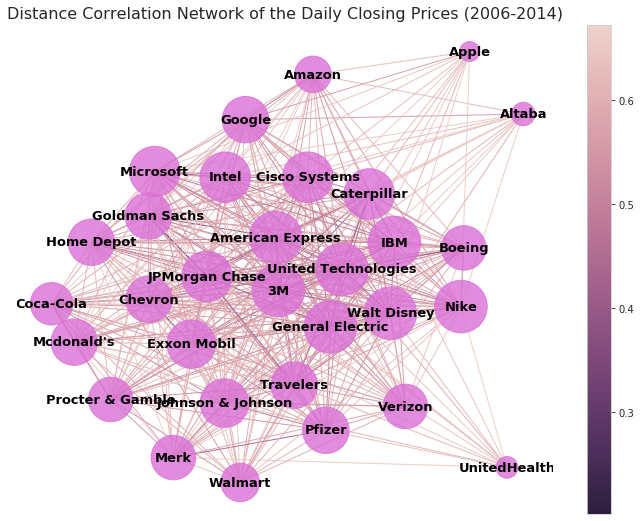In the above visualization, the sizes of the vertices are proportional to the number of connections they have. The colorbar to the right indicates the degree of disimilarity (the distance) between the stocks. The larger the value (the lighter the color) the less similar the stocks are. Keeping this in mind, several stocks jump out. Apple, Amazon, Altaba, and UnitedHealth all lie on the periphery of the network with the fewest number of correlations above $\rho_{c} = 0.325$. On the other hand 3M, American Express, United Technolgies, and General Electric sit in the core of the network with the greatest number connections above $\rho_{c} = 0.325$. It is clear from the closing prices network that our asset allocation algorithm needs to reward vertices on the periphery and punish those nearing the center. In the next code block we build a function to visualize how the edges of the distance correlation network are distributed.

## Degree Histogram¶

In :
# function to visualize the degree distribution
def hist_plot(network, title, bins, xticks):

# extracts the degrees of each vertex and stores them as a list
deg_list = list(dict(network.degree).values())

# sets local style
with plt.style.context('fivethirtyeight'):
# initializes a figure
plt.figure(figsize=(9,6))

# plots a pretty degree histogram with a kernel density estimator
sns.distplot(
deg_list,
kde=True,
bins = bins,
color='darksalmon',
hist_kws={'alpha': 0.7}

);

# turns the grid off
plt.grid(False)

# controls the number and spacing of xticks and yticks
plt.xticks(xticks, size=11)
plt.yticks(size=11)

# removes the figure spines
sns.despine(left=True, right=True, bottom=True, top=True)

# labels the y and x axis
plt.ylabel("Probability", size=15)
plt.xlabel("Number of Connections", size=15)

# sets the title
plt.title(title, size=20);

# draws a vertical line where the mean is
plt.axvline(sum(deg_list)/len(deg_list),
color='darkorchid',
linewidth=3,
linestyle='--',
label='Mean = {:2.0f}'.format(sum(deg_list)/len(deg_list))
)

# turns the legend on
plt.legend(loc=0, fontsize=12)

In :
# plots the degree histogram of the closing prices network
hist_plot(
H_close,
'Degree Histogram of the Closing Prices Network',
bins=9,
xticks=range(13, 30, 2)
)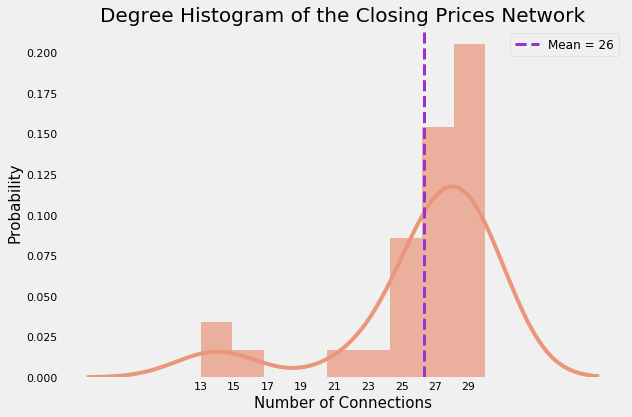Observations

• The degree distribution is left-skewed.
• The average node is connected to 86.6% of the network.
• Very few nodes are connected to less than 66.6% of the network.
• The kernel density estimation is not a good fit.
• By eyeballing the plot, the degrees appear to follow an inverse power-law distribution. (This would be consistent with the findings of Tse, et al. (2010)).
In :
plt_corr_nx(
H_close_diff,
title='Distance Correlation Network of the Daily Net Change in Price (2006-2014)'
)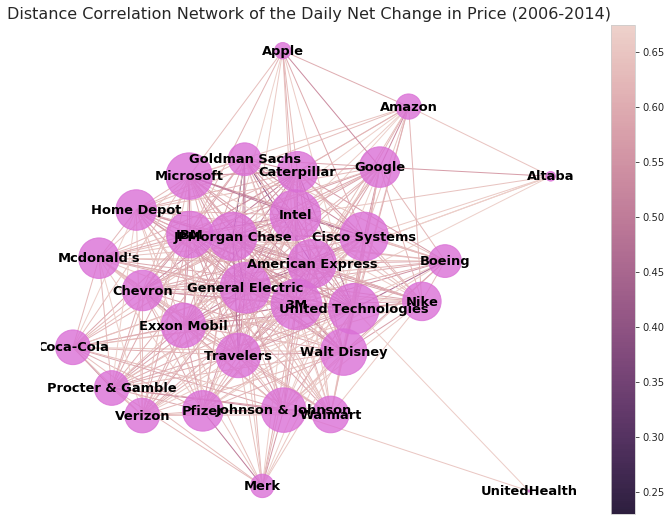Observations

• The above network has substantially fewer edges than the former.
• Apple, Amazon, Altaba, UnitedHealth, and Merck have the fewest number of correlations above $\rho_{c}$.
• 3M, General Electric, American Express, Walt Disney, and United Technologies have the greatest number of correlations above $\rho_{c}$.
• UnitedHealth is clearly an outlier with only two connections above $\rho_{c}$.
In :
hist_plot(
H_close_diff,
'Degree Histogram of the Daily Net Change in Price Network',
bins=9,
xticks=range(2, 30, 2)
)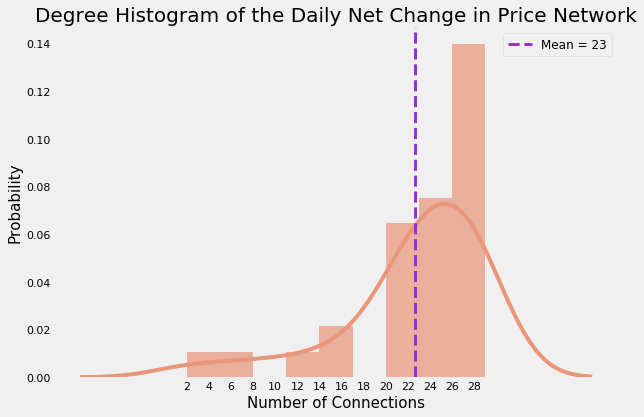Observations:

• The distribution is left-skewed.
• The average node is connected to 73.3% of the network.
• Very few nodes are connected to less than 53.3% of the network.
• The kernel density estimation is a poor fit.
• The degree distribution appears to follow an inverse power-law.
In :
plt_corr_nx(
H_high,
title='Distance Correlation Network of the Daily High Prices (2006-2014)'
)